# Quasi-Frobenius ring

QF-ring

A (left or right) Artinian ring satisfying the annihilator conditions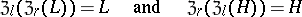for each left (or right) ideal(respectively,) (see Annihilator). A left Artinian ring that satisfies only one of these annihilator conditions need not be a quasi-Frobenius ring. Quasi-Frobenius rings are of interest because of the presence of duality: A left Artinian ringis a quasi-Frobenius ring if and only if the mapping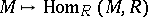defines a duality between the categories of left and right finitely-generated-modules. A finite-dimensional algebraover a fieldis a quasi-Frobenius ring if and only if each irreducible right summand of the left-module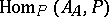is isomorphic to some minimal left ideal of. And this is equivalent to the self-duality of the lattices of left and right ideals of.

Quasi-Frobenius rings were introduced as a generalization of Frobenius algebras, determined by the requirement that the right and left regular representations are equivalent. For a left and right Artinian ringthe property of being quasi-Frobenius was originally defined in the following way: If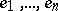is the complete list of primitive idempotents of(that is,for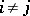, and for any primitive idempotent,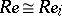for some),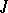is the radical ofand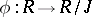is the natural homomorphism, then there is a permutationof the set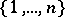such that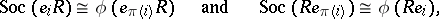where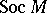is the socle of the module. The property ofbeing quasi-Frobenius is equivalent also to each of the following properties: 1)is left Noetherian (cf. Noetherian ring),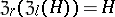for every right idealand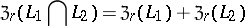for any left ideals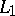and; 2)satisfies the maximum condition for left (or right) annihilator ideals (in particular, ifis left and right Noetherian) and is left and right self-injective (cf. Self-injective ring); 3)is right Artinian and left and right self-injective; 4) every injective (projective) left-module is projective (injective) (cf. Projective module; Injective module); 5) every flat left-module is injective (cf. Flat module); 6)is left and right self-injective and right perfect (cf. Perfect ring); 7)is left and right self-injective and each of its right ideals is an annihilator of some finite set in; 8)is right perfect and every finitely-generated left-module is contained in a projective module; 9)is coherent (cf. Coherent ring), right perfect, andfor all finitely-presented left-modules; 10)satisfies the maximum condition for left annihilators and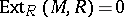for all finitely-presented left-modules; 11)is left and right Artinian and for every finitely-generated left-modulethe lengths of the modulesand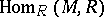are the same; 12) the ring of endomorphisms of each free left-module is left self-injective; or 13) finitely-generated one-sided ideals of the ring of endomorphisms of a projective generator (injective co-generator) of the category of left-modules are annihilators.

Injective modules over a quasi-Frobenius ring split into a direct sum of cyclic modules. For commutative rings the converse is also true. If the Jacobson radicalof a ringis transfinitely nilpotent (that is,for some transfinite number, where,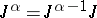and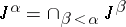for a limit ordinal number), thenis a quasi-Frobenius ring if and only ifis left self-injective and all its one-sided ideals are annihilators. A left module over a quasi-Frobenius ringis faithful if and only if it is a generator of the category of left-modules. The group ring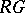is a quasi-Frobenius ring and if and only ifis a finite group andis a quasi-Frobenius ring.

Certain generalizations of quasi-Frobenius rings have also been studied; a left QF--ringis defined by the requirement that there exists a faithful left-module that is contained as a direct summand in any faithful left-module; a left QF--ringis defined by the requirement that the injective hull of the left-modulecan be imbedded in the direct product of some set of copies of. A left pseudo-Frobenius ring (or left PF-ring) is defined by each of the following properties: a)is an injective co-generator of the category of left-modules; b) every faithful left-module is a generator of the category of left-modules; or c)is a left QF--ring and the annihilator of any right ideal different fromis non-zero.